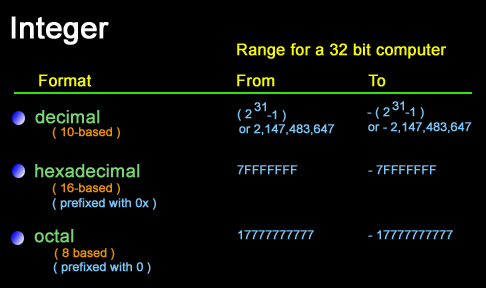# PHP Data Types:  Integers and Floating point numbers

## PHP Integers

An integer must have at least one digit (0-9). No comma or blanks are allowed within an integer.

It must not have a decimal point.

It could be either positive or negative if no sign precedes it is assumed to be positive.

Integers can be specified in three formats, decimal (10-based), hexadecimal (16-based) (prefixed with 0x) or octal (8-based) (prefixed with 0) notation, optionally preceded by a - or + sign.Example :

``````<?php
\$x = 4356; //decimal number
echo \$x.' is : ';
var_dump(\$x);
\$x = -39; //a negative number
echo '<br />'.\$x.' is : ';
var_dump(\$x);
\$x = 057; //octal number (equivalent to 47 decimal)
echo '<br />'.\$x.' is : ';
var_dump(\$x);
\$x = 0x4B; //hexadecimal number (equivalent to 75
//decimal)
echo '<br />'.\$x.' is : ';
var_dump(\$x);
?>``````

View the example in browser

Output:

```4356 is : int(4356)
-39 is : int(-39)
47 is : int(47)
75 is : int(75)```

Note: var_dump() function is used to get structured information (type and value) about variables.

PHP integers: Range

The size and range of an integer are platform depended. For a 32 bit computer maximum number the largest integer is 231-1 or 2,147,483,647 and the smallest integer is -(231-1) or -2,147,483,647. if we specify a number beyond the range of the integer type, it will be interpreted as a float. Integer size can be determined from PHP_INT_SIZE, maximum value from PHP_INT_MAX. If you specify a number beyond the bounds of the integer type, it will be interpreted as a float instead.

Floating point numbers

Description

Floating point numbers could be written in two forms, fractional and exponential form.

Fractional form :

The number must have at least one digit (0-9). No comma or blanks are allowed within an integer. It must have a decimal point. It could be either positive or negative, default sign is positive.

Exponential form :

The exponent part is an "e" or "E" followed by an integer, which can be signed (preceded by "+" or "-").

Version

(PHP 4 and above)

Example:

``````<?php
\$a = 5.205;
\$b = 1.3e3;
\$c = 6E-10;
?>``````

Previous: Booleans
Next: Strings

﻿

## PHP: Tips of the Day

PHP: How to convert string to boolean?

Strings always evaluate to boolean true unless they have a value that's considered "empty" by PHP (taken from the documentation for empty):

1. "" (an empty string);
2. "0" (0 as a string)

If you need to set a boolean based on the text value of a string, then you'll need to check for the presence or otherwise of that value.

`\$test_mode_mail = \$string === 'true'? true: false;`

EDIT: the above code is intended for clarity of understanding. In actual use the following code may be more appropriate:

`\$test_mode_mail = (\$string === 'true');`

or maybe use of the filter_var function may cover more boolean values:

`filter_var(\$string, FILTER_VALIDATE_BOOLEAN);`

filter_var covers a whole range of values, including the truthy values "true", "1", "yes" and "on".

Ref : https://bit.ly/33SQ3eP